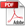# Application Developer's Guide — Chapter 13

## Function Values

This chapter describes how to use function values, which allow you to pass function values as parameters to XQuery functions. It includes the following sections:

### Overview of Function Values

XQuery functions take parameters, and those parameters can be any XQuery type. Typically, parameters are strings, dates, numbers, and so on, and XQuery has many types to provide robust typing support. Sometimes, however, it is convenient to pass a pointer to a named function as a parameter to another function. These function pointers are known as function values, and they allow you to write code that can be more robust and more easily maintainable. Programming languages that support passing functions as parameters sometimes call those higher order functions. MarkLogic Server function values do most things that higher order functions in other languages do, except you cannot output a function and you cannot create anonymous functions; instead, you can output or input a function value, which is implemented as an XQuery primitive type.

You pass a function value to another function by telling it the name of the function you want to pass. The actual value returned by the function is evaluated dynamically during query runtime. Passing these function values allows you to define an interface to a function and have a default implementation of it, while allowing callers of that function to implement their own version of the function and specify it instead of the default version.

### xdmp:function XQuery Primitive Type

Function values are defined as an xdmp:function XQuery primitive type. You can use this type in function or variable definitions, or in the same way as you use other primitive types in XQuery. Unlike some of the other MarkLogic Server XQuery primitive types (`cts:query` and map:map, for example), there is no XML serialization for the xdmp:function XQuery primitive type.

### XQuery APIs for Function Values

The following XQuery built-in functions are used to pass function values:

You use xdmp:function to specify the function to pass in, and xdmp:apply to run the function that is passed in. For details and the signature of these APIs, see the MarkLogic XQuery and XSLT Function Reference.

### When the Applied Function is an Update from a Query Statement

When you apply a function using xdmp:function, MarkLogic Server does not know the contents of the applied function at query compilation time. Therefore, if the statement calling xdmp:apply is a query statement (that is, it contains no update expressions and therefore runs at a timestamp), and the function being applied is performing an update, then it will throw an `XDMP-UDATEFUNCTIONFROMQUERY` exception.

If you have code that you will apply that performs an update, and if the calling query does not have any update statements, then you must make the calling query an update statement. To change a query statement to be an update statement, either use the `xdmp:update` prolog option or put an update call somewhere in the statement. For example, to force a query to run as an update statement, you can add the following to your XQuery prolog:

`declare option xdmp:update "true";`

Without the prolog option, any update expression in the query will force it to run as an update statement. For example, the following expression will force the query to run as an update statement and not change anything else about the query:

```if ( fn:true() )
then ()
else xdmp:document-insert("fake.xml", <fake/>)```

For details on the difference between update statements and query statements, see Understanding Transactions in MarkLogic Server.

### Example of Using Function Values

The following example shows a recursive function, `my:sum:sequences`, that takes an xdmp:function type, then applies that function call recursively until it reaches the end of the sequence. It shows how the caller can supply her own implementation of the `my:add` function to change the behavior of the `my:sum-sequences` function. Consider the following library module named `/sum.xqy`:

```xquery version "1.0-ml";
module namespace my="my-namespace";

(: Sum a sequence of numbers, starting with the
starting-number (3rd parameter) and at the
start-position (4th parameter). :)
declare function my:sum-sequence(
\$fun as xdmp:function,
\$items as item()*,
\$starting-number as item(),
\$start-position as xs:unsignedInt)
as item()
{
if (\$start-position gt fn:count(\$items)) then \$starting-number
else
let \$new-value := xdmp:apply(\$fun,\$starting-number,
\$items[\$start-position])
return
my:sum-sequence(\$fun,\$items,\$new-value,\$start-position+1)
};

declare function my:add(\$x,\$y) {\$x+ \$y};
(: /sum.xqy :)```

Now call this function with the following main module:

```xquery version "1.0-ml";
import module namespace my="my-namespace" at "/sum.xqy";

let \$fn := xdmp:function(xs:QName("my:add"))
return my:sum-sequence(\$fn,(1 to 100), 2, 1)```

This returns 5052, which is the sum of all of the numbers between 2 and 100.

If you want to use a different formula for adding up the numbers, you can create an XQuery library module with a different implementation of the same function and specify it instead. For example, assume you want to use a different formula to add up the numbers, and you create another library module named `/my.xqy` that has the following code (it multiplies the second number by two before adding it to the first):

```xquery version "1.0-ml";
module namespace my="my-namespace";

declare function my:add(\$x,\$y) {\$x+ (2 * \$y)};
(: /my.xqy :)```

You can now call the `my:sum-sequence` function specifying your new implementation of the `my:add` function as follows:

```xquery version "1.0-ml";
import module namespace my="my-namespace" at "/sum.xqy";

let \$fn := xdmp:function(xs:QName("my:add"), "/my.xqy")
return my:sum-sequence(\$fn,(1 to 100), 2, 1)```

This returns 10102 using the new formula. This technique makes it possible for the caller to specify a completely different implementation of the specified function that is passed.

« Previous chapter
Next chapter »